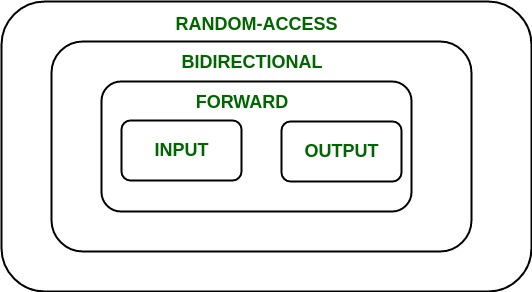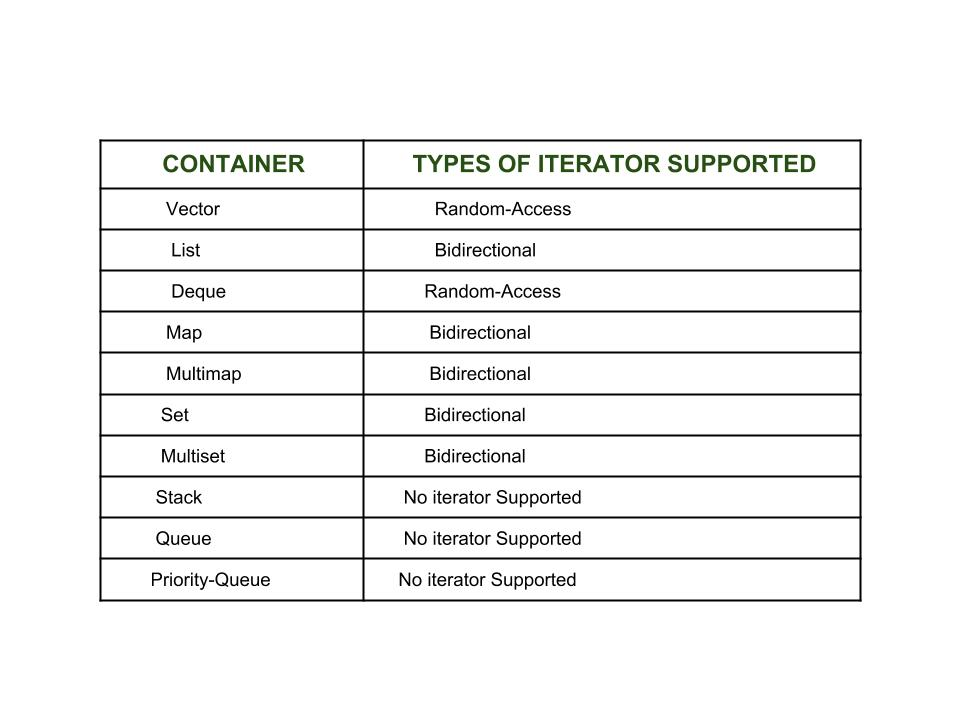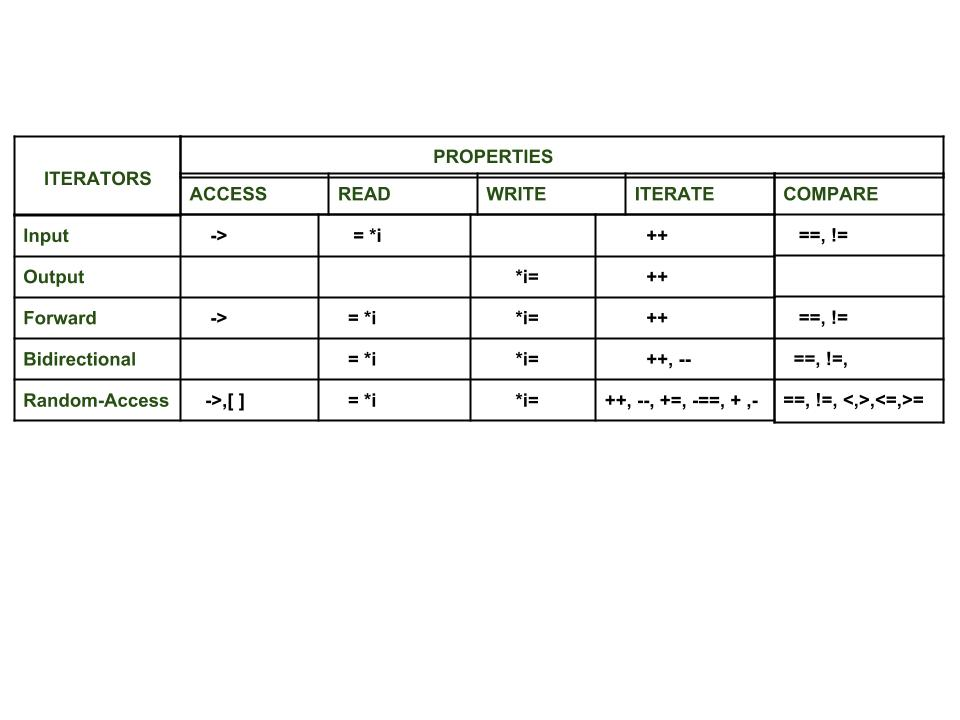Related Articles

# Introduction to Iterators in C++

• Difficulty Level : Medium
• Last Updated : 26 May, 2020

An iterator is an object (like a pointer) that points to an element inside the container. We can use iterators to move through the contents of the container. They can be visualized as something similar to a pointer pointing to some location and we can access the content at that particular location using them.

Iterators play a critical role in connecting algorithm with containers along with the manipulation of data stored inside the containers. The most obvious form of an iterator is a pointer. A pointer can point to elements in an array and can iterate through them using the increment operator (++). But, all iterators do not have similar functionality as that of pointers.

Depending upon the functionality of iterators they can be classified into five categories, as shown in the diagram below with the outer one being the most powerful one and consequently the inner one is the least powerful in terms of functionality.Now each one of these iterators are not supported by all the containers in STL, different containers support different iterators, like vectors support Random-access iterators, while lists support bidirectional iterators. The whole list is as given below:Types of iterators: Based upon the functionality of the iterators, they can be classified into five major categories:

1. Input Iterators: They are the weakest of all the iterators and have very limited functionality. They can only be used in a single-pass algorithms, i.e., those algorithms which process the container sequentially, such that no element is accessed more than once.
2. Output Iterators: Just like input iterators, they are also very limited in their functionality and can only be used in single-pass algorithm, but not for accessing elements, but for being assigned elements.
3. Forward Iterator: They are higher in the hierarachy than input and output iterators, and contain all the features present in these two iterators. But, as the name suggests, they also can only move in a forward direction and that too one step at a time.
4. Bidirectional Iterators: They have all the features of forward iterators along with the fact that they overcome the drawback of forward iterators, as they can move in both the directions, that is why their name is bidirectional.
5. Random-Access Iterators: They are the most powerful iterators. They are not limited to moving sequentially, as their name suggests, they can randomly access any element inside the container. They are the ones whose functionality are same as pointers.

The following diagram shows the difference in their functionality with respect to various operations that they can perform.Benefits of Iterators

There are certainly quite a few ways which show that iterators are extremely useful to us and encourage us to use it profoundly. Some of the benefits of using iterators are as listed below:

1. Convenience in programming: It is better to use iterators to iterate through the contents of containers as if we will not use an iterator and access elements using [ ] operator, then we need to be always worried about the size of the container, whereas with iterators we can simply use member function end() and iterate through the contents without having to keep anything in mind.
 `// C++ program to demonstrate iterators`` ` `#include ``#include ``using` `namespace` `std;``int` `main()``{``    ``// Declaring a vector``    ``vector<``int``> v = { 1, 2, 3 };`` ` `    ``// Declaring an iterator``    ``vector<``int``>::iterator i;`` ` `    ``int` `j;`` ` `    ``cout << ``"Without iterators = "``;``     ` `    ``// Accessing the elements without using iterators``    ``for` `(j = 0; j < 3; ++j) ``    ``{``        ``cout << v[j] << ``" "``;``    ``}`` ` `    ``cout << ``"\nWith iterators = "``;``     ` `    ``// Accessing the elements using iterators``    ``for` `(i = v.begin(); i != v.end(); ++i)``    ``{``        ``cout << *i << ``" "``;``    ``}`` ` `    ``// Adding one more element to vector``    ``v.push_back(4);`` ` `    ``cout << ``"\nWithout iterators = "``;``     ` `    ``// Accessing the elements without using iterators``    ``for` `(j = 0; j < 4; ++j) ``    ``{``        ``cout << v[j] << ``" "``;``    ``}`` ` `    ``cout << ``"\nWith iterators = "``;``     ` `    ``// Accessing the elements using iterators``    ``for` `(i = v.begin(); i != v.end(); ++i) ``    ``{``        ``cout << *i << ``" "``;``    ``}`` ` `    ``return` `0;``}`

Output:

```Without iterators = 1 2 3
With iterators = 1 2 3
Without iterators = 1 2 3 4
With iterators = 1 2 3 4
```

Explanation: As can be seen in the above code that without using iterators we need to keep track of the total elements in the container. In the beginning there were only three elements, but after one more element was inserted into it, accordingly the for loop also had to be amended, but using iterators, both the time the for loop remained the same. So, iterator eased our task.

2. Code reusability: Now consider if we make v a list in place of vector in the above program and if we were not using iterators to access the elements and only using [ ] operator, then in that case this way of accessing was of no use for list (as they don’t support random-access iterators).

However, if we were using iterators for vectors to access the elements, then just changing the vector to list in the declaration of the iterator would have served the purpose, without doing anything else
So, iterators support reusability of code, as they can be used to access elements of any container.

3. Dynamic processing of the container: Iterators provide us the ability to dynamically add or remove elements from the container as and when we want with ease.
 `// C++ program to demonstrate iterators`` ` `#include ``#include ``using` `namespace` `std;``int` `main()``{``    ``// Declaring a vector``    ``vector<``int``> v = { 1, 2, 3 };`` ` `    ``// Declaring an iterator``    ``vector<``int``>::iterator i;`` ` `    ``int` `j;`` ` `    ``// Inserting element using iterators``    ``for` `(i = v.begin(); i != v.end(); ++i) {``        ``if` `(i == v.begin()) {``            ``i = v.insert(i, 5);``            ``// inserting 5 at the beginning of v``        ``}``    ``}``     ` `    ``// v contains 5 1 2 3`` ` `    ``// Deleting a element using iterators``    ``for` `(i = v.begin(); i != v.end(); ++i) {``        ``if` `(i == v.begin() + 1) {``            ``i = v.erase(i);``            ``// i now points to the element after the``            ``// deleted element``        ``}``    ``}``     ` `    ``// v contains 5 2 3`` ` `    ``// Accessing the elements using iterators``    ``for` `(i = v.begin(); i != v.end(); ++i) {``        ``cout << *i << ``" "``;``    ``}`` ` `    ``return` `0;``}`

Output:

```5 2 3
```

Explanation: As seen in the above code, we can easily and dynamically add and remove elements from the container using iterator, however, doing the same without using them would have been very tedious as it would require shifting the elements every time before insertion and after deletion.

This article is contributed by Mrigendra Singh. If you like GeeksforGeeks and would like to contribute, you can also write an article using contribute.geeksforgeeks.org or mail your article to contribute@geeksforgeeks.org. See your article appearing on the GeeksforGeeks main page and help other Geeks.